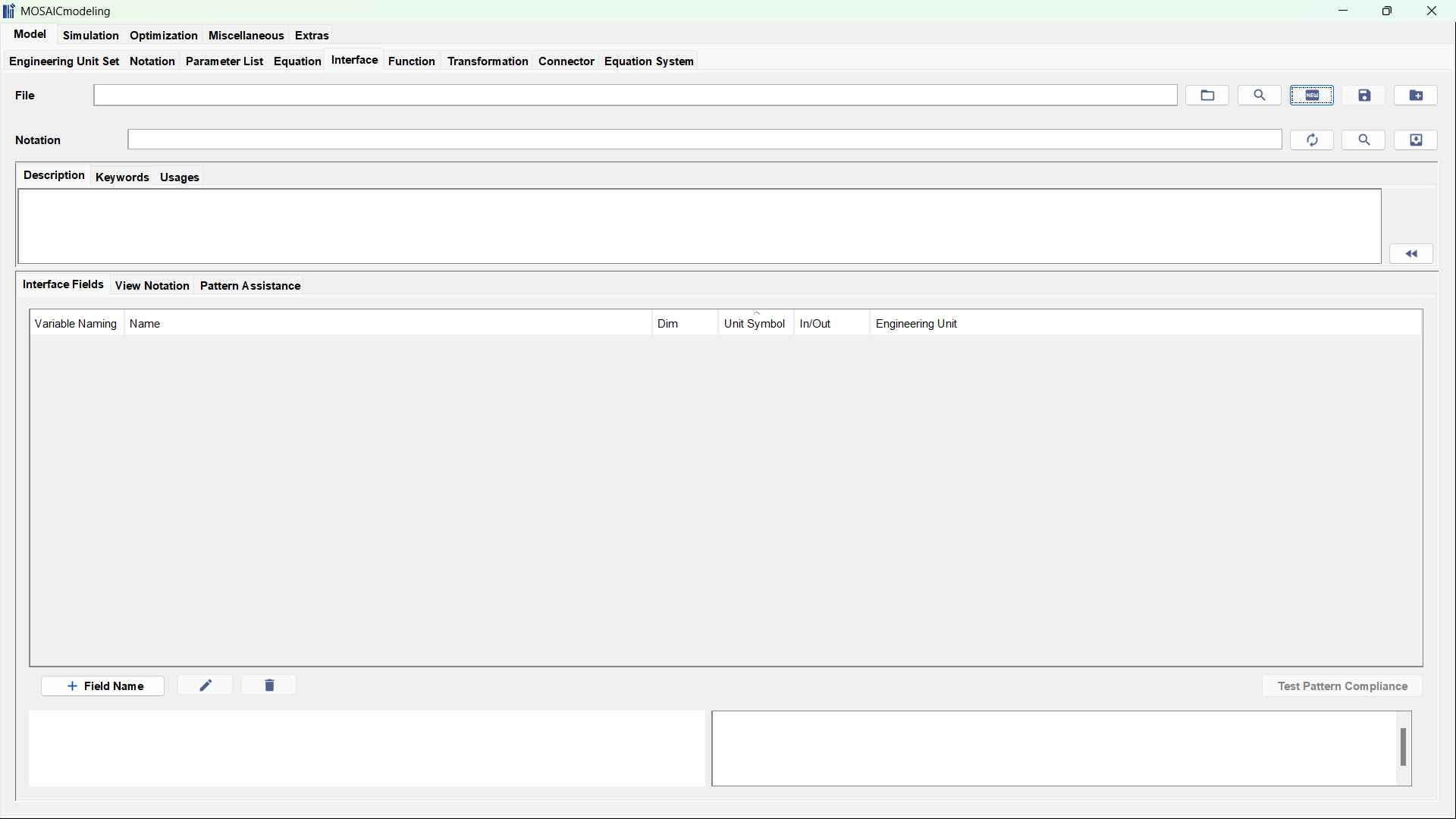# Model: Interface

Optional element

Modeling physical phenomena with descriptive equations also requires the use of well defined variable sets to make sure that the units within the equations are correct and to avoid errors. An Interface for a chemical engineering unit could contain the variables$F$,$z_c$,$P$,$T$, and$w$. The notation for such an interface would explain$F$ as molar feed stream,$z_c$ as molar fraction of the composition (phase independent),$P$ as pressure,$T$ as temperature, and$w$ as vapor fraction.

### Purpose

Interfaces standardize communication between model elements, e.g., when

• connecting different process units via streams
• making calls to external functions

They define what information can be exchanged, specifically

• variable name
• description
• dimension (scalar or vector)
• direction (in or out or in/out)
• engineering unit

Note that they do not represent the actual external function, but only provide the interface. This is discussed further in functions.

### Explanation of the editor

Figure 1 shows the interface editor whereas Table 1 explains the entries of the interface editor.Figure 1: The interface editor lets you define interfaces that can, for example, be used for external function calls.